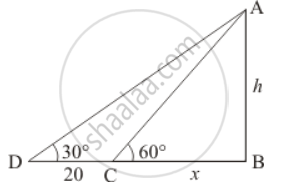Share

# A T.V. Tower Stands Vertically on a Bank of a River. from a Point on the Other Bank Directly Opposite the Tower, the Angle of Elevation of the Top of the Tower is 60°. from a Point 20 M Away this Point on the Same Bank, the Angle of Elevation of the Top of the Tower is 30°. Find the Height of the Tower and the Width of the River. - CBSE Class 10 - Mathematics

#### Question

A T.V. Tower stands vertically on a bank of a river. From a point on the other bank directly opposite the tower, the angle of elevation of the top of the tower is 60°. From a point 20 m away this point on the same bank, the angle of elevation of the top of the tower is 30°. Find the height of the tower and the width of the river.

#### Solution

Let AB be the T.V tower of height hm on a bank of river and D be the point on the opposite of the river. An angle of elevation at top of the tower is 60° and from a point 20m away then an angle of elevation of the tower at the same point is 30°. Let AB = h and BC = x.

Here we have to find height and width of the river.

The corresponding figure is hereIn  ΔCAB

=> tan 60^@ = (AB)/(BC)

=> sqrt3 = h/x

=> sqrt3x = h

=> x = h/sqrt3

Again in ΔDBA

=> tan 30^@ = (AB)/(BC)

=> 1/sqrt3 = h/(20 + x)

=> sqrt3h = 20 + x

=> sqrt3h = 20 + h/sqrt3

=> sqrt3h - h/sqrt3 = 20

=> (2h)/sqrt3 = 20

=> h = 10sqrt3

=> x = (10sqrt3)/sqrt3

=> x = 10

Hence the height of the tower is 10sqrt3 m and width of rived is  10 m

Is there an error in this question or solution?

#### Video TutorialsVIEW ALL 

Solution A T.V. Tower Stands Vertically on a Bank of a River. from a Point on the Other Bank Directly Opposite the Tower, the Angle of Elevation of the Top of the Tower is 60°. from a Point 20 M Away this Point on the Same Bank, the Angle of Elevation of the Top of the Tower is 30°. Find the Height of the Tower and the Width of the River. Concept: Heights and Distances.
S